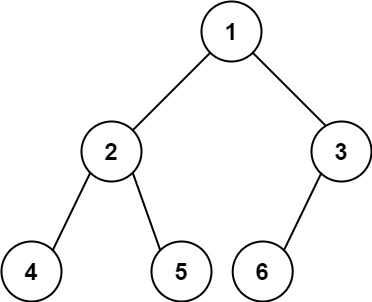# GeetCode Hub

Given the `root` of a complete binary tree, return the number of the nodes in the tree.

According to Wikipedia, every level, except possibly the last, is completely filled in a complete binary tree, and all nodes in the last level are as far left as possible. It can have between `1` and `2h` nodes inclusive at the last level `h`.

Design an algorithm that runs in less than `O(n)` time complexity.

Example 1:```Input: root = [1,2,3,4,5,6]
Output: 6
```

Example 2:

```Input: root = []
Output: 0
```

Example 3:

```Input: root = 
Output: 1
```

Constraints:

• The number of nodes in the tree is in the range `[0, 5 * 104]`.
• `0 <= Node.val <= 5 * 104`
• The tree is guaranteed to be complete.

/** * Definition for a binary tree node. * public class TreeNode { * int val; * TreeNode left; * TreeNode right; * TreeNode() {} * TreeNode(int val) { this.val = val; } * TreeNode(int val, TreeNode left, TreeNode right) { * this.val = val; * this.left = left; * this.right = right; * } * } */ class Solution { public int countNodes(TreeNode root) { } }